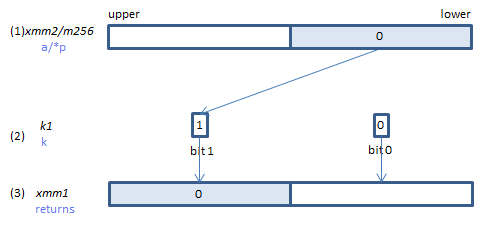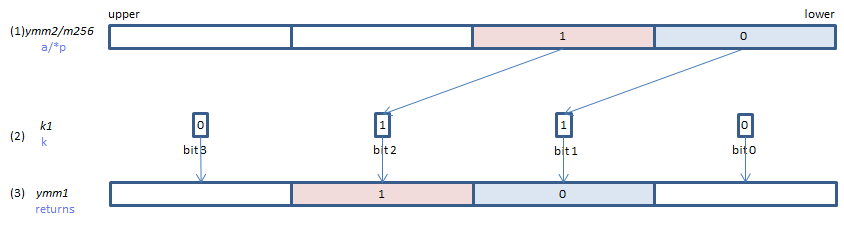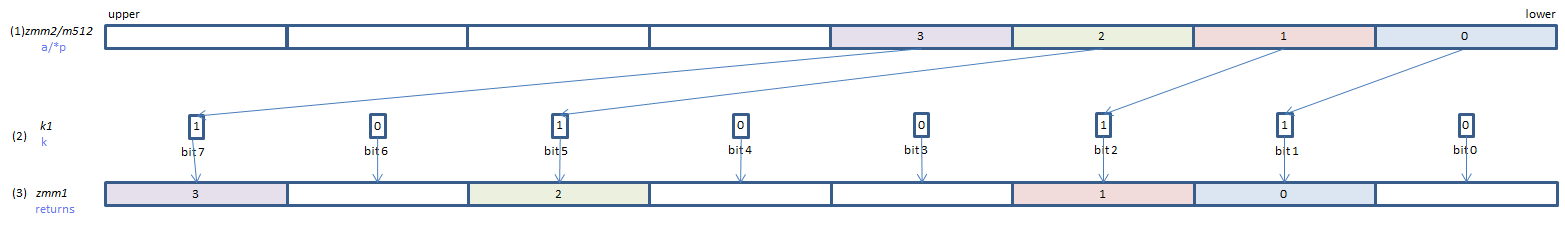﻿ vexpandpd

## VEXPANDPD - EXPAND Packed Double

VEXPANDPD xmm1{k1}{z}, xmm2/m128    (V5+VLIf each bit of (2) is set, the corresponding element of (3) is copied from (1), taken one by one from the lowest element.
If corresponding bit of (2) is not set, (3) element is:
zero cleared if {z} is specified (_maskz_ intrinsic is used)
left unchanged if {z} is not specified. (copied from s if _mask_ intrinsic is used.)
VEXPANDPD ymm1{k1}{z}, ymm2/m256    (V5+VLIf each bit of (2) is set, the corresponding element of (3) is copied from (1), taken one by one from the lowest element.
If corresponding bit of (2) is not set, (3) element is:
zero cleared if {z} is specified (_maskz_ intrinsic is used)
left unchanged if {z} is not specified. (copied from s if _mask_ intrinsic is used.)
VEXPANDPD zmm1{k1}{z}, zmm2/m512    (V5